# Right triangles

How many right triangles we can construct from line segments 3,4,5,6,8,10,12,13,15,17 cm long? (Do not forget to the triangle inequality).

Correct result:

n =  4

#### Solution:

Δ 3 4 5
Δ 5 12 13
Δ 6 8 10
Δ 8 15 17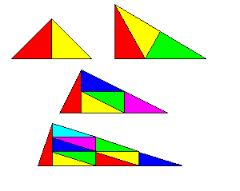We would be pleased if you find an error in the word problem, spelling mistakes, or inaccuracies and send it to us. Thank you!Tips to related online calculators
Pythagorean theorem is the base for the right triangle calculator.

#### You need to know the following knowledge to solve this word math problem:

We encourage you to watch this tutorial video on this math problem:

## Next similar math problems:

• Triangles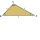Five sticks with a length of 2,3,4,5,6 cm. How many ways can you choose three sticks to form three sides of a triangle?
• Triangles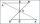Hanka cut the 20 cm long straws into three pieces each piece had a length in cm. Then, with these three pieces, she tried to make a triangle. a) What circuit has each of the triangles? b) How long can the longest side measure? c) How many different triang
• Sides od triangleSides of the triangle ABC has length 4 cm, 5 cm and 7 cm. Construct triangle A'B'C' that are similar to triangle ABC which has a circumference of 12 cm.
• TriangleProve whether you can construct a triangle ABC, if a=9 cm, b=6 cm, c=10 cm.
• TrianglesIvo wants to draw all the triangles whose two sides of which have a length of 4 cm and 9 cm and the length of the third side is expressed in whole centimeters. How many triangles does he have?
• Circle tangentIt is given to a circle with the center S and radius 3.5 cm. Distance from the center to line p is 6 cm. Construct a circle tangent n which is perpendicular to the line p.
• Tangent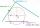What distance is the tangent t of the circle (S, 4 cm) and the chord of this circle, which is 6 cm long and parallel to the tangent t?
• Right angled triangleHypotenuse of a right triangle is 17 cm long. When we decrease length of legs by 3 cm then decrease its hypotenuse by 4 cm. Determine the size of legs.
• Construct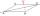Construct a rhombus ABCD, if the size of the diagonal AC is 6 cm and diagonal BD 8 cm long.
• Isosceles trapezoidCalculate the content of an isosceles trapezoid whose bases are at ratio 5:3, the arm is 6cm long and it is 4cm high.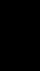The cone-shaped lampshade has a diameter of 30 cm and a height of 10 cm. How many cm2 of material will we need when we 10% is waste?There is regular hexagon ABCDEF. If area of the triangle ABC is 22, what is area of the hexagon ABCDEF? I do not know how to solve it simply....The rectangle, one side of which is 5 cm long, is divided by a 13 cm diagonal into two triangles. Calculate the area of one of these triangles in cm2.The base of the prism is a regular hexagon consisting of six triangles with side a = 12 cm and height va = 10.4 cm. The prism height is 5 cm. Find the volume and surface of the prism.The lengths of the cuboid edges are in the ratio 1: 2: 3. Will the lengths of its diagonals be the same ratio? The cuboid has dimensions of 5 cm, 10 cm, and 15 cm. Calculate the size of the wall diagonals of this cuboid.Line segments 62 cm and 2.2 dm long we divide into equal parts which lengths in centimeters is expressed integer. How many ways can we divide?Construct rhombus ABCD if given diagonal length | AC | = 8cm, inscribed circle radius r = 1.5cm